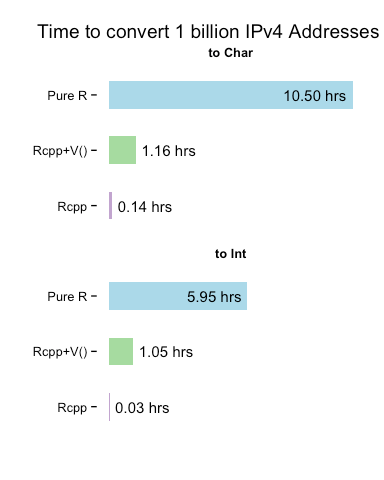Want to share your content on R-bloggers? click here if you have a blog, or here if you don't.

The previous
post

looked at using the `Vectorize()` function to, well, vectorize, our
Rcpp IPv4
functions
.
While this is a completely acceptable practice, we can perform the
vectorization 100% in `Rcpp`/C++. We’ve included both the original
`Rcpp` IPv4 functions and the new `Rcpp`-vectorized functions together
to show the minimal differences between them:

```#include
#include

using namespace Rcpp;
using namespace boost::asio::ip;

// Rcpp/C++ vectorized routines

// [[Rcpp::export]]
NumericVector rcpp_rinet_pton (CharacterVector ip) {

int ipCt = ip.size(); // how many elements in vector

NumericVector ipInt(ipCt); // allocate new numeric vector

// CONVERT ALL THE THINGS!
for (int i=0; i
We’ve merely wrapped a `for` loop around the original code and built the
result vectors in `Rcpp`, relying on the object-oriented nature of C++
for proper value conversion+assignment. The pure-R+`Vectorize()`‘d code
(from the examples in the book) is below, since
# Vectorize() the single-element vector routines
v_rinet_pton <- Vectorize(rinet_pton, USE.NAMES=FALSE)
v_rinet_ntop <- Vectorize(rinet_ntop, USE.NAMES=FALSE)

# pure R version with Vectorize()
ip2long <- Vectorize(function(ip) {
ips <- unlist(strsplit(ip, '.', fixed=TRUE))
octet <- function(x,y) bitOr(bitShiftL(x, 8), y)
Reduce(octet, as.integer(ips))
}, USE.NAMES=FALSE)

long2ip <- Vectorize(function(longip) {
octet <- function(nbits) bitAnd(bitShiftR(longip, nbits), 0xFF)
paste(Map(octet, c(24,16,8,0)), sep="", collapse=".")
}, USE.NAMES=FALSE)

Now, we’ll read in a file of ~8,000 IPv4 addresses, make them into
integers and then use the `microbenchmark` package to profile the
to/from conversion of all three versions of the routines.
# read in ~8K IP address strings & make ints for our benchmark
ints <- rcpp_rinet_pton(ips\$V1)

# run a benchmark 100 times per routine, giving plenty of "ramp up" time
mb <- microbenchmark(rcpp_ints <- rcpp_rinet_pton(ips\$V1),
rcpp_chars <- rcpp_rinet_ntop(ints),
v_ints <- v_rinet_pton(ips\$V1),
v_chars <- v_rinet_ntop(ints),
r_ints <- ip2long(ips\$V1),
r_chars <- long2ip(ints),
control=list(warmup=20),
times=100, unit="s")

Then, we’ll take a look at the results (all times are in seconds):

td { font-family:monospace; font-size:0.8em; padding:5px} table { margin-bottom:12px;}

Version

min

lq

median

uq

max

Rcpp-toInt

0.0007216090

0.0007610835

0.0007967235

0.0008572075

0.0026142800

Rcpp-toChar

0.0037574850

0.0038886490

0.0039565840

0.0040140285

0.0046188840

Rcpp+V()-toInt

0.0217142230

0.0266931380

0.0290988580

0.0316722610

0.0775550730

Rcpp+V()-toChar

0.0253528670

0.0290143845

0.0322646160

0.0346684450

0.0814177860

Pure R-toInt

0.1480684080

0.1588533500

0.1654142360

0.1701886530

0.1992565150

Pure R-toChar

0.2726176440

0.2863672665

0.2917557870

0.2960467515

0.3371749450

If we just look at the median values, we can see that the conversion
to integer takes:

Version

median

Rcpp-toInt

0.0007967235

Rcpp+V()-toInt

0.0290988580

Pure R-toInt

0.1654142360

and, the conversion to character takes:

Version

median

Rcpp-toChar

0.0039565840

Rcpp+V()-toChar

0.0322646160

Pure R-toChar

0.2917557870

But, a visualization is (often) worth a dozen tables, so we’ll take the
test results and make a violin plot (which is just a more granular
boxplot). Note that the plot is on a log scale, so the differences
between each set of comparisons are actually much larger than your eye
will initially comprehend (hence the inclusion of the above tables).It’s often difficult for us to grok fractional seconds, so let’s do some
basic math to see how long each method would take to process 1
billion IP addresses. We’ll use the median values from above and
compare the results in a simple bar chart:The fully vectorized `Rcpp` versions are the clear “winners” and will let
us scale our IPv4 address conversions to millions, billions or trillions
of operations without having to rely on other scripting languages. We
can use this base as foundation for a complete IP address `S4` class
that we’ll cover in future posts.
You can find the `Rmd` source that helped generate this post over at github along with the data file.

var vglnk = {key: '949efb41171ac6ec1bf7f206d57e90b8'};
(function(d, t) {
var s = d.createElement(t);
s.type = 'text/javascript';
s.async = true;
// s.defer = true;
var r = d.getElementsByTagName(t);
r.parentNode.insertBefore(s, r);
}(document, 'script'));

Related
ShareTweet